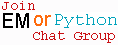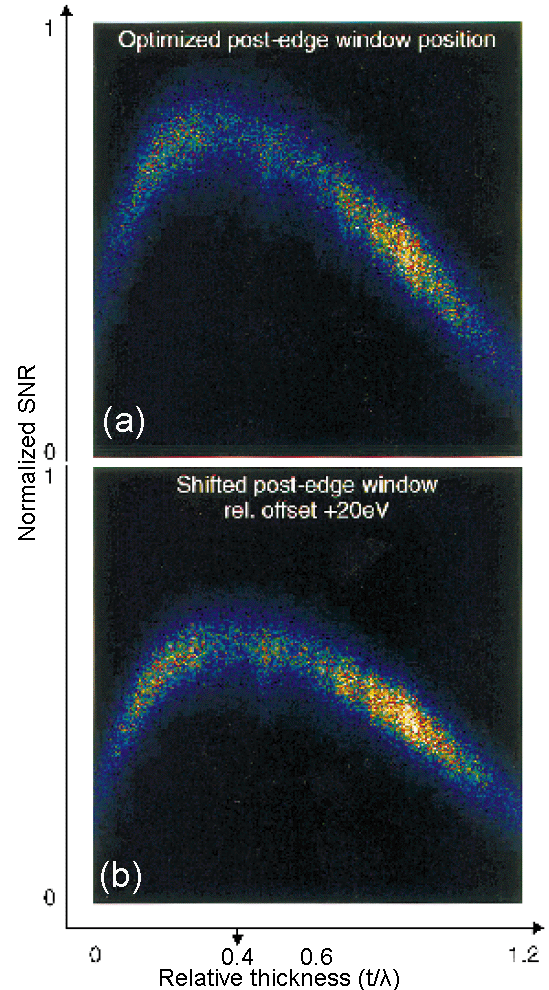Mass-Thickness Effects on Measured EELS Signal/Intensity
- Practical Electron Microscopy and Database -
- An Online Book -Microanalysis | EM Book                                                                   http://www.globalsino.com/EM/

Figure 1385a shows two correlation plots calculated from filtered image series taken with a 25 eV slit at conditions in three window method: pre-edge 1 = 225 eV, pre-edge 2 = 265 eV and with post-edge = 295 eV and with energy window = 315 – 295 = 20 eV, respectively. The optimal specimen thickness around t/λ = 0.4 for carbon under the condition of 200 keV (energy of the incident electron beam) and 7.6 mrad (collection angle). Extremely thin specimen areas cause the SNR (Signal-to-Noise Ratio) to drop due to increased noise, whereas plural scattering in thick areas decreases the signal relative to the background (similar to the case described in Figure 1385b). A shift of the post-edge window by 20 eV (Figure 1385a (b)) lowers the maximum SNR value of approximately 25% as a fact of a larger background extrapolation error (h) and a decreased signal.Figure 1385a. Correlation plots showing the influence of thickness and of changes in post-edge window position on the signal to noise ratio .

The major drawbacks and difficulty in quantitative and even qualitative EELS analysis is the strong effect of the sample thickness on the detection of EELS edges. For instance, the spectrum from "thick" TEM sample might show no edges. Figure 1385b shows that the background increases dramatically with increase of the thickness of TEM specimen, while the signal of Pt M4,5 edge decreases significantly. The highest signal in the range of these TEM specimen thicknesses for Pt occurs at 20 nm.Figure 1385b. EELS of Pt M4,5 edge.

Furthermore, as presented by Hawkes and Spence , this severe limitation to the visibility of edges is due to the contribution of multiple inelastic scattering that increases the background under the edge. On the other hand, the contribution of multiple scattering is not constant as a function of energy loss and the quantification of spectra for increasingly thick samples demonstrates a variation of the apparent concentration with thickness. Measurements of the ratio of two elements, for instance, show that samples with thickness relative to the mean inelastic free path t/λ > 0.5 are unreliable (Figure 1385c). Even when multiple scattering effects are removed with deconvolution techniques, the effects still persist , indicating that additional contributions due to the angular distributions of losses are present and cannot be neglected in correction approaches.Figure 1385c. Variation in the quantification of two elements as a function of thickness relative to the total inelastic mean free path. 

It also shows, see page3819, that there is an angular dependence of thickness effect on visibility of EELS singal.

Figure 1385d shows the mass-thickness effects on both EDS and EELS signals (or intensities). Both signals increases with the mass-thickness factor (f) when the factor f is smaller than fms1, while the the EELS signal decreases rapidly when f is greater than fms1. On the other hand, the EELS signal becomes smaller than EDS signal when f is greater than fms2. Therefore, the EDS and EELS signals will be reversed between the cases of f < fms1 and f >fms2. That is, in the case of f >fms2, higher concentration of an specific element probably does not provide high intensity or contrast in EELS measurements, but it does for EDS.Figure 1385d. Mass-thickness effects on both EDS and EELS signals (or intensities).

 Optimization of the signal to noise ratio in EFTEM elemental maps with regard to different eonization edge types, Kothleitner, G and Hofer, F, Micron, 29, 5, pp. 349–357, (1998).
 Peter W. Hawkes and John C.H. Spence, Science of Microscopy, Volume I, (2007).
 Egerton, R. (Ed.) (1996). Electron Energy-Loss Spectroscopy in the Electron Microscope, 2nd ed. (Plenum, New York).

 ;